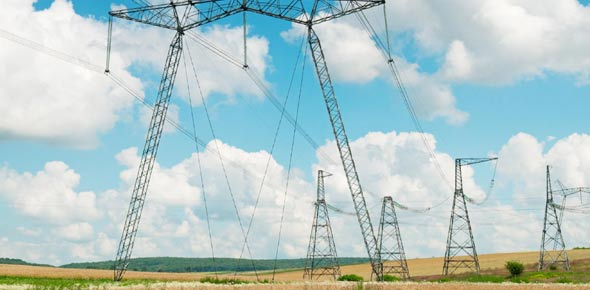10 Questions | Total Attempts: 251SettingsElectricity can be defined as the flow of electric charge, but that's not enough. Do you really know how electricity is generated? And from where does it come? There are a number of questions unanswered. This quiz has been developed for students, researcher and learners to gain and test their knowledge about electricity. So, let's try out the quiz. All the best!

• 1.
When electricity is flowing in a complete path that is called ______________ circuit.
• A.

Closed

• B.

Open

• C.

Light bulb

• 2.
When electricity is not flowing that is called a ___________ circuit.
• A.

Hammer

• B.

Open

• C.

Closed

• D.

Sun

• E.

Moon

• 3.
Does a light bulb light up with a gap in it _______?
• A.

Yes

• B.

No

• 4.
Two particles with the same charge will repel or drive away from each other
• A.

True

• B.

False

• 5.
When one has connected a conductor, a source of electricity such as a battery and an appliance such as a bulb, one has created an electric
• A.

Insulator

• B.

Circuit

• C.

Switch

• D.

None of the above

• 6.
This is the measure of electric current.
• A.

Ohm

• B.

Ampere

• C.

Volt

• D.

Coulomb

• 7.
This measures resistance to the flow of electricity.
• A.

Ohm

• B.

Ampere

• C.

Volt

• D.

Coulomb

• 8.
This device is used to open and close a circuit.
• A.

Battery

• B.

Bulb

• C.

Switch

• D.

None of the above

• 9.
The positive terminals or ends of the batteries and appliances are wired together and the negative ends of batteries and appliances are wired together in this kind of circuit
• A.

Short

• B.

Series

• C.

Parallel

• D.

None of the above

• 10.
These materials conduct electricity under certain circumstances
• A.

Insulators

• B.

Conductor

• C.

Semiconductor

• D.

None of the above

Related TopicsBack to top